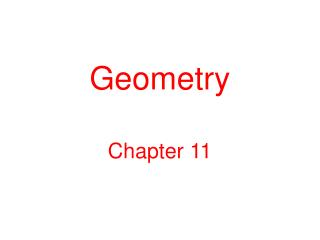DownloadDownload PresentationGeometry

# Geometry

Download Presentation## Geometry

- - - - - - - - - - - - - - - - - - - - - - - - - - - E N D - - - - - - - - - - - - - - - - - - - - - - - - - - -
##### Presentation Transcript

1. Geometry Chapter 11

2. InformalStudy of Shape • Until about 600 B.C. geometry was pursued in response to practical, artistic and religious needs. Considerable knowledge of geometry was accumulated, but mathematics was not yet an organized and independent discipline. • Beginning in about 600 B.C. Pythagoras, Euclid, Thales, Zeno, Eudoxus and others began organizing the knowledge accumulated by experience and transformed geometry into a theoretical science. • NOTE that the formality came only AFTER the informality of experience in practical, artistic and religious settings! • In this class, we return to learning by trusting our intuition and experience. We will discover by exploring using picture representations and physical models.

3. Informal Study ofShape • Shape is an undefined term. • New shapes are being discovered all the time. • FRACTALS

4. InformalStudyof Shape Our goals are: • To recognize differences and similarities among shapes • To analyze the properties of a shape or class of shapes • To model, construct and draw shapes in a variety of ways.

5. NCTM Standard Geometry in Grades Pre-K-2 Children begin forming concepts of shape long before formal schooling. They recognize shape by its appearance through qualities such as “pointiness.” They may think that a shape is a rectangle because it “looks like a door.” Young children begin describing objects by talking about how they are the same or how they are different. Teachers will then help them to gradually incorporate conventional terminology. Children need many examples and nonexamples to develop and refine their understanding. The goal is to lay the foundation for more formal geometry in later grades.

6. Point • Line • Collinear • Plane

7. If two lines intersect, their intersection is a point, called the point of intersection. • Parallel Lines

8. Concurrent

9. Skew Lines – nonintersecting lines that are not parallel.

10. Line segment • Endpoint • Length

11. Congruent

12. Midpoint

13. Half Line • A point separates a line into 3 disjoint sets: The point, and 2 half lines.

14. Ray - the union of a half line and the point.

15. Angle – the union of two rays with a common endpoint.

16. The angle separates the plane into 3 disjoint sets: The angle, the interior of the angle, and the exterior of the angle.

17. Degrees • Protractor

18. Zero Angle: 0° • Straight Angle: 180° • Right Angle: 90°

19. Acute Angle: between 0° and 90° • Obtuse Angle: between 90° and 180°

20. Reflex Angle

21. Perpendicular Lines

22. Adjacent Angles

23. Adjacent Angles

24. Vertical Angles

25. Vertical Angles

26. The sum of the measures of Complementary Angles is 90°.

27. Complementary angles • Adjacent complementary angles

28. The sum of the measures of Supplementary Angles is 180°.

29. Supplementary Angles • Adjacent Supplementary Angles

30. Transversal • Corresponding Angles

31. Transversal • Corresponding Angles

32. Parallel lines Cut by a Transversal

33. Parallel lines Cut by a Transversal • Corresponding Angles

34. Parallel lines Cut by a Transversal • Corresponding Angles

35. Describe the relative position of angles 3 and 5. • What appears to be true about their measures?

36. Alternate Interior Angles

37. Describe the relative positions of angles 1 and 7. • What appears to be true about their measures?

38. Alternate Exterior Angles

39. Triangle

40. The sum of the measure of the interior angles of any triangle is 180°.

41. Exterior Angle

42. The measure of the exterior angle of a triangle is equal to the sum of the measure of the two opposite interior angles.

43. Note: Homework Page 672 #37

44. DAY 2

45. Homework QuestionsPage 667

46. #16